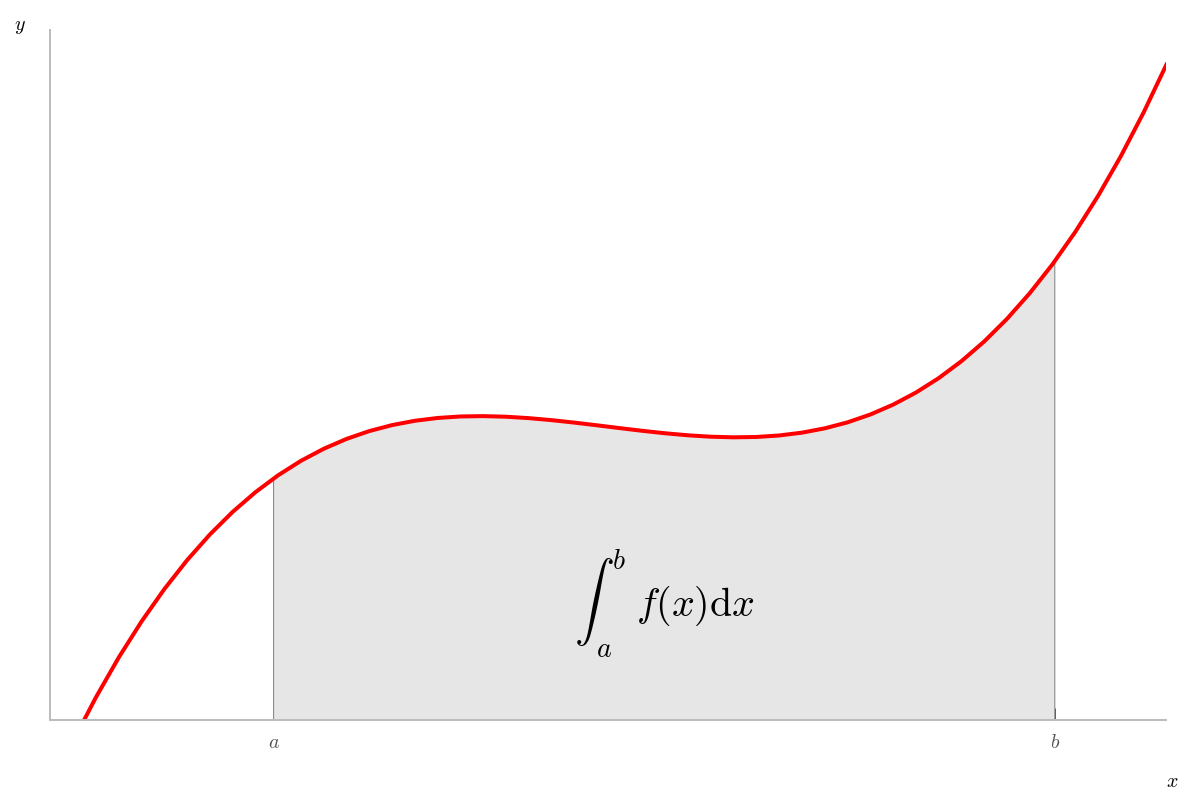# Working With External Code¶

The IPython Kernel makes it easy to incorporate external code from sources such as the internet or copy/paste.

## Pasting code into cells¶

You can copy and paste code from other sources directly into cells. Pasting code with >>> prompts works as expected:

In :
>>> the_world_is_flat = 1
>>> if the_world_is_flat:
...     print("Be careful not to fall off!")

Be careful not to fall off!


## The %load magic¶

The %load magic lets you load code from URLs or local files:

In :
%load?

In :
%matplotlib inline

In [ ]:
%load http://matplotlib.org/mpl_examples/showcase/integral_demo.py

In :
# %load http://matplotlib.org/mpl_examples/showcase/integral_demo.py
"""
Plot demonstrating the integral as the area under a curve.

Although this is a simple example, it demonstrates some important tweaks:

* A simple line plot with custom color and line width.
* A shaded region created using a Polygon patch.
* A text label with mathtext rendering.
* figtext calls to label the x- and y-axes.
* Use of axis spines to hide the top and right spines.
* Custom tick placement and labels.
"""
import numpy as np
import matplotlib.pyplot as plt
from matplotlib.patches import Polygon

def func(x):
return (x - 3) * (x - 5) * (x - 7) + 85

a, b = 2, 9 # integral limits
x = np.linspace(0, 10)
y = func(x)

fig, ax = plt.subplots()
plt.plot(x, y, 'r', linewidth=2)
plt.ylim(ymin=0)

# Make the shaded region
ix = np.linspace(a, b)
iy = func(ix)
verts = [(a, 0)] + list(zip(ix, iy)) + [(b, 0)]
poly = Polygon(verts, facecolor='0.9', edgecolor='0.5')
ax.add_patch(poly)

plt.text(0.5 * (a + b), 30, r"$\int_a^b f(x)\mathrm{d}x$",
horizontalalignment='center', fontsize=20)

plt.figtext(0.9, 0.05, '$x$')
plt.figtext(0.1, 0.9, '$y$')

ax.spines['right'].set_visible(False)
ax.spines['top'].set_visible(False)
ax.xaxis.set_ticks_position('bottom')

ax.set_xticks((a, b))
ax.set_xticklabels(('$a$', '$b$'))
ax.set_yticks([])

plt.show()